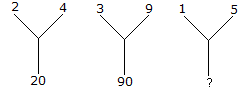# Verbal Reasoning - Character Puzzles - Discussion

### Discussion :: Character Puzzles - Character Puzzles 1 (Q.No.38)

38.

Which one will replace the question mark ?[A]. 20 [B]. 26 [C]. 25 [D]. 75

Explanation:

(2)2 + (4)2 = 20

(3)2 + (9)2 = 90

Therefore, (1)2 + (5)2 = 26.

 Souvik said: (Aug 1, 2018) 4 * 5 = 20, 9 * 10 = 90, so, 5 * 15 = 75.

 Aryampa said: (Aug 21, 2021) Thanks @Souvik.